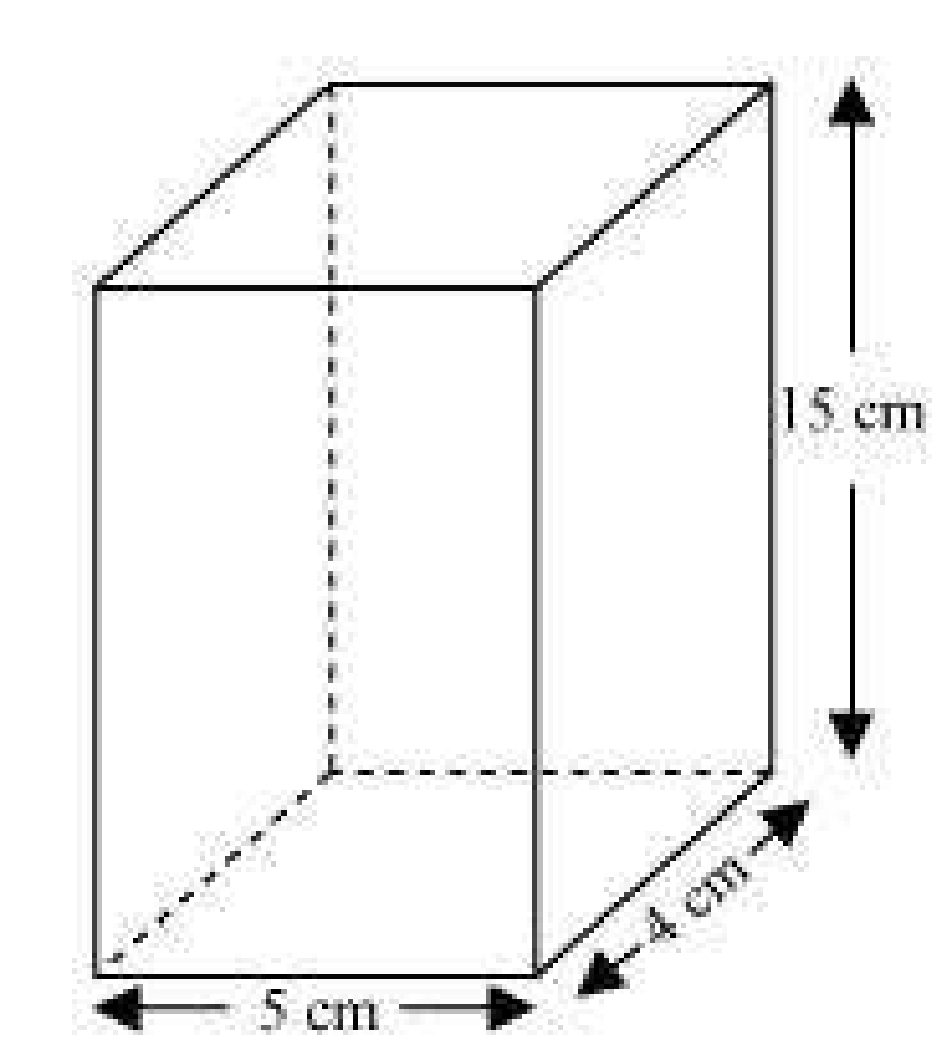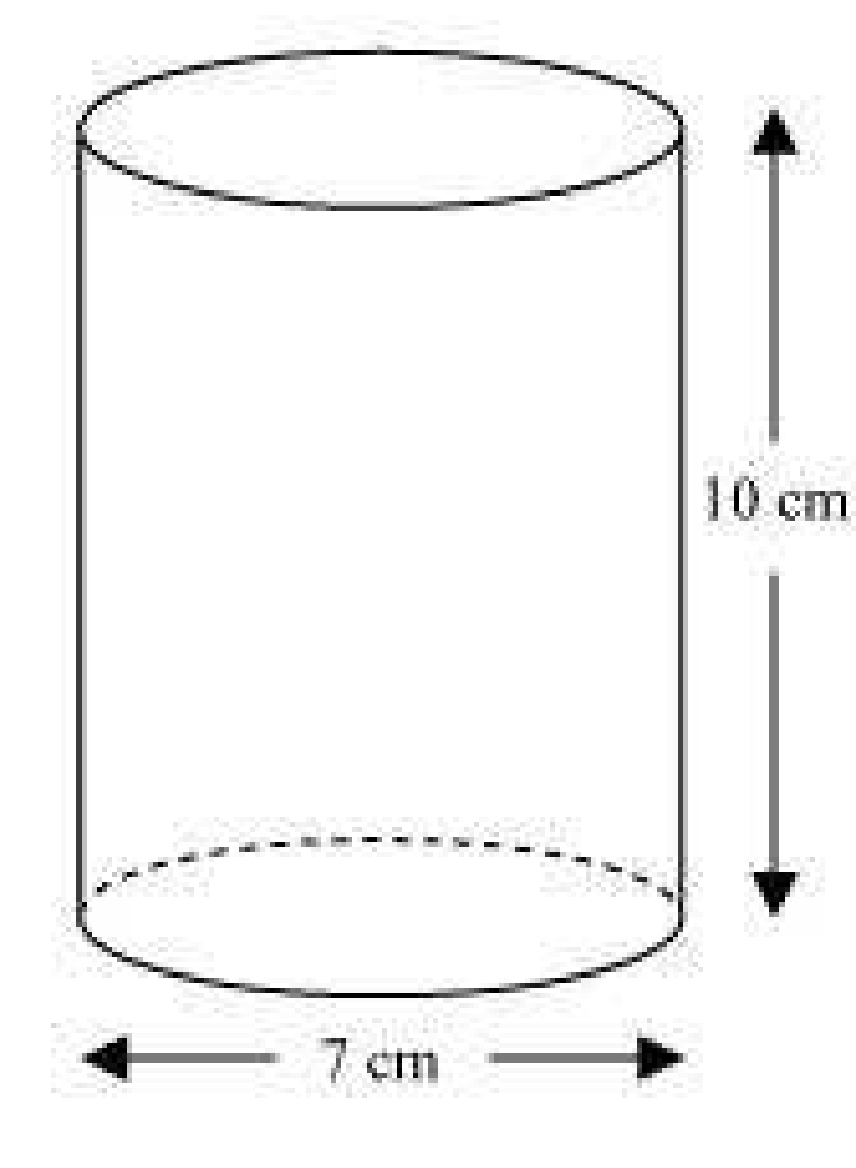# A soft drink is available in two packs - (i) a tin can with a rectangular base of length 5cm and width 4cm, having a height of 15 cm and (ii) a plastic cylinder with circular base of diameter 7cm and height 10cm. Which container has greater capacity and by how much? (Assume π = 22/7)

Solution

(i) tin can will be cuboidal in shapeLength of can (l) = 5 cm

Width of can (b) = 4 cm

Height of can (h) = 15 cm

So,

Length of can (l) = 5 cm

Width of can (b) = 4 cm

Height of can (h) = 15 cm

The amount of soft drink it can hold = volume of cuboid

= (l × b × h)

= 5 × 4 × 15 cm3

= 300 cm3

(l × b × h)

= 5 × 4 × 15 cm3

= 300 cm3

(ii) Plastic cylinder will be cylindrical in shape.Radius of can (r) =cm

Height of can (h) = 10 cm

The amount of soft drink it can hold = volume of Cylinder= (πr2h)

= 22/7 X 7/2 X 7/2 X 10 cm3

= 385 cm3

Difference in capacity = (385-300) cm3 = 85cm3

Tin can that has circular base can contain 85cm3 more amount of soft drinks than the first can.